## Basketball and soccer are played with a spherical ball. Even though an athlete dribbles the ball in both sports, a basketball player uses his hands and a soccer player uses his feet. Usually, soccer is played outdoors on a large field and basketball is played indoor on a court made out of wood. The projectile (path traced) of soccer ball and basketball are in the form of parabola representing quadratic polynomial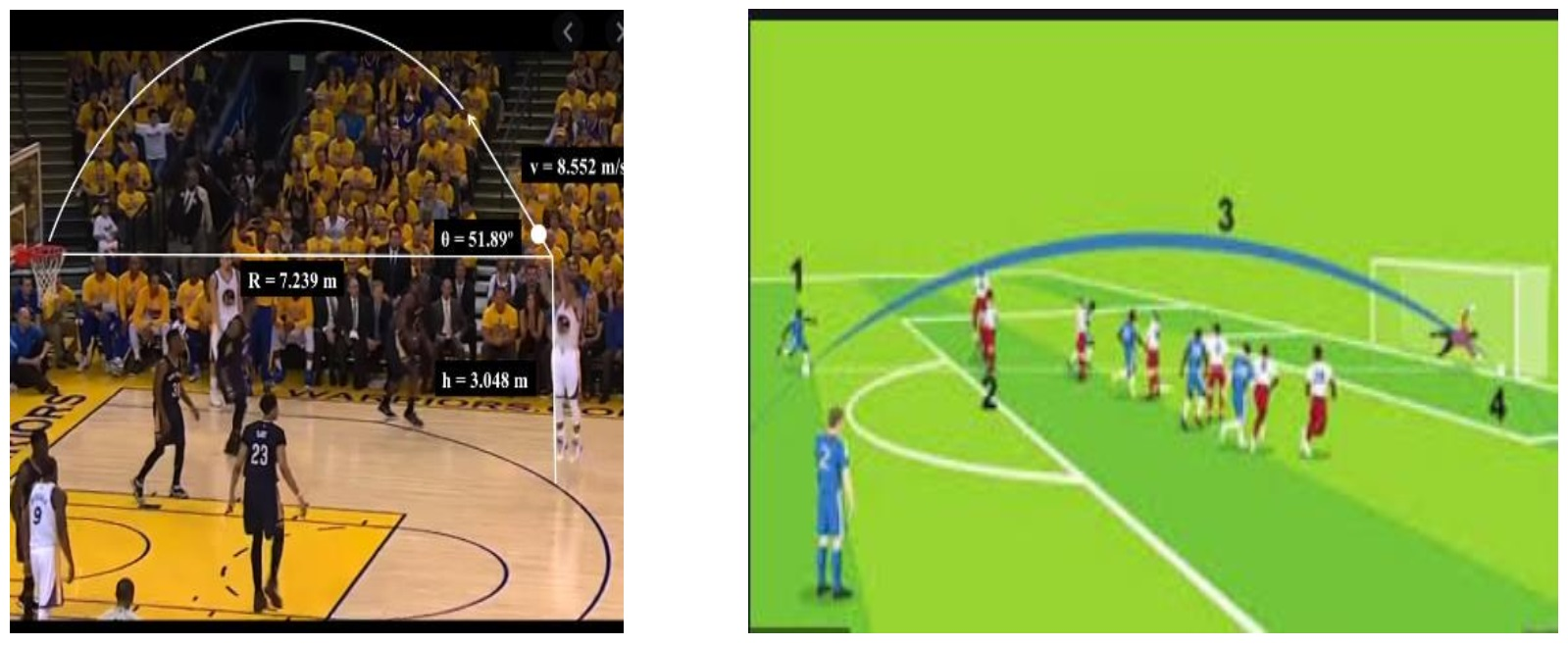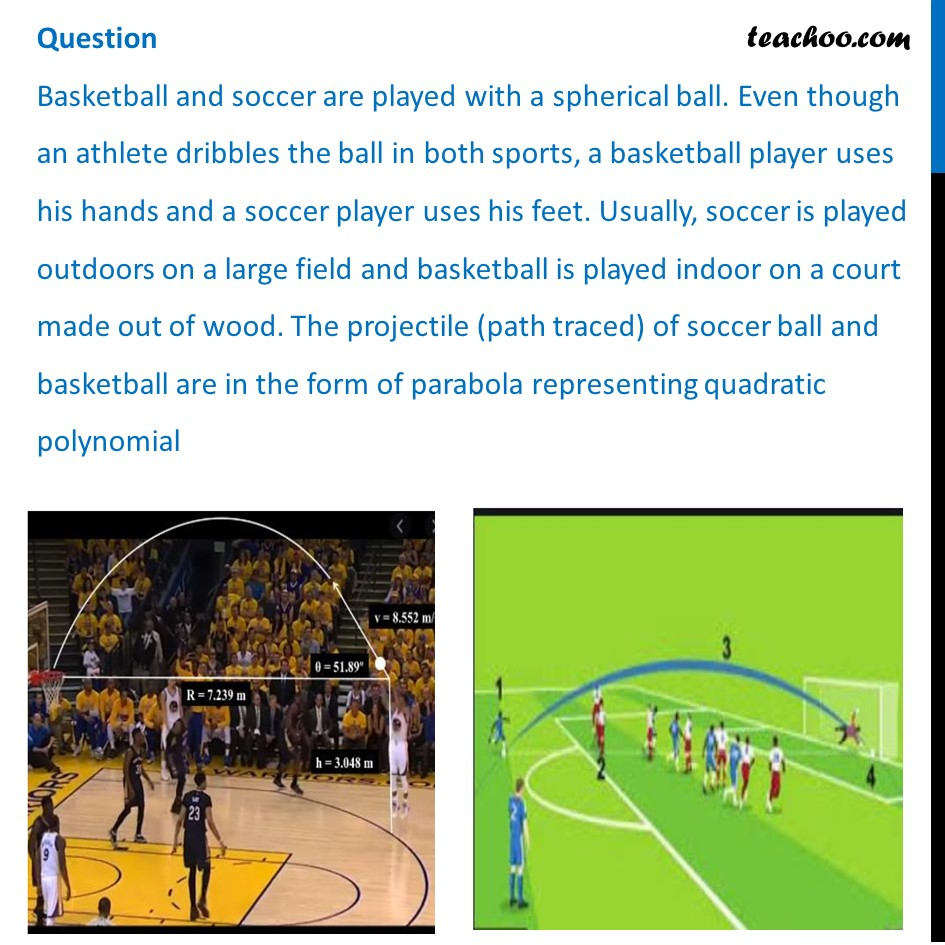## The shape of the path traced shown is (a) Spiral  (b) Ellipse  (c) Linear  (d) Parabola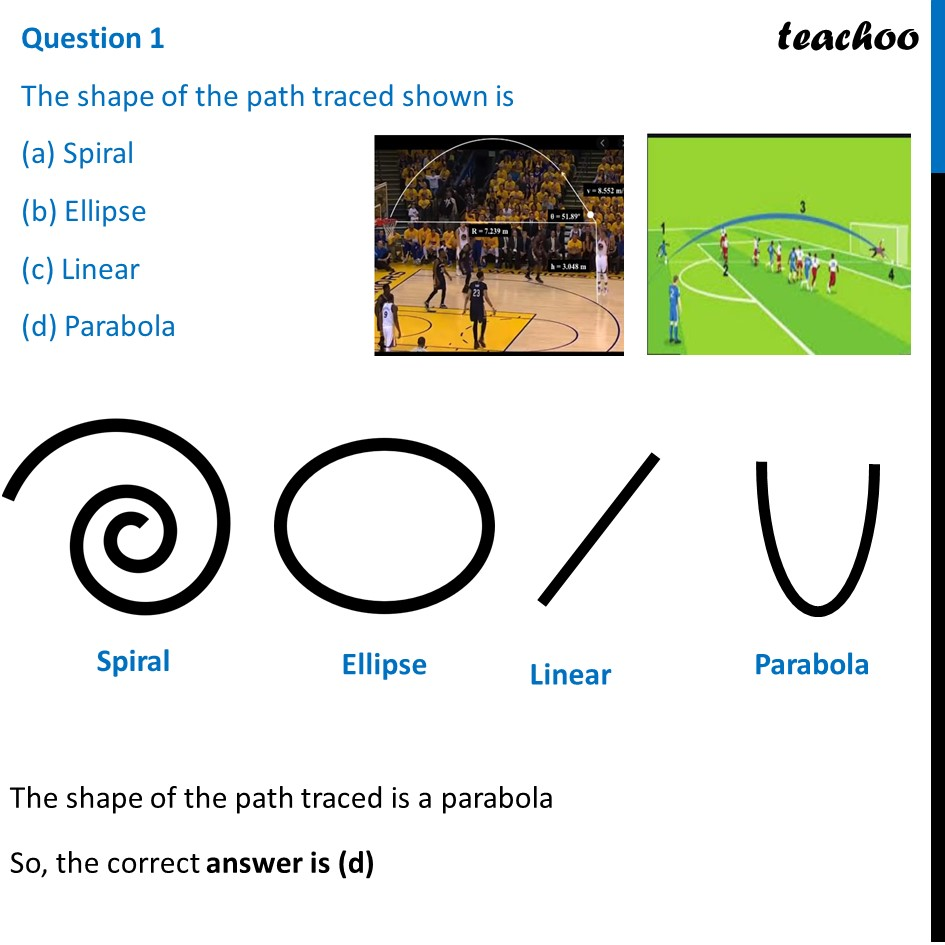## The graph of parabola opens upwards, if _______ (a) a = 0   (b) a < 0   (c) a > 0   (d) a ≥ 0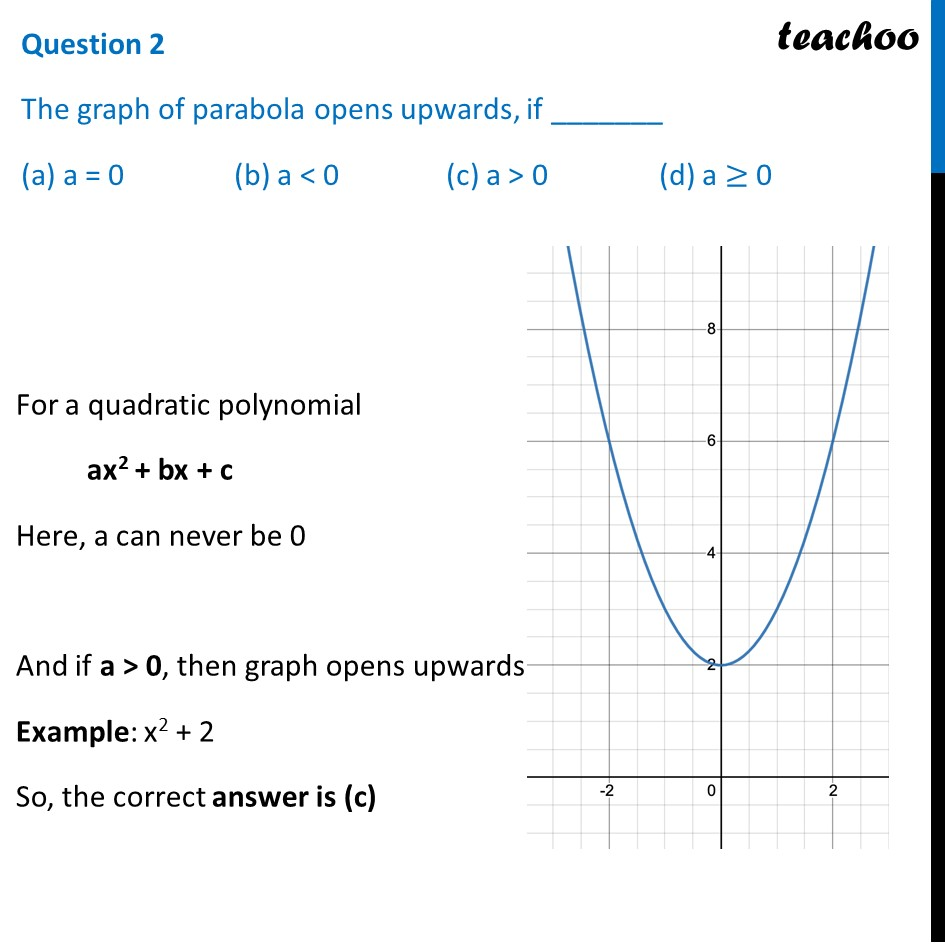## Observe the following graph and answer In the above graph, how many zeroes are there for the polynomial? (a) 0  (b) 1  (c) 2  (d) 3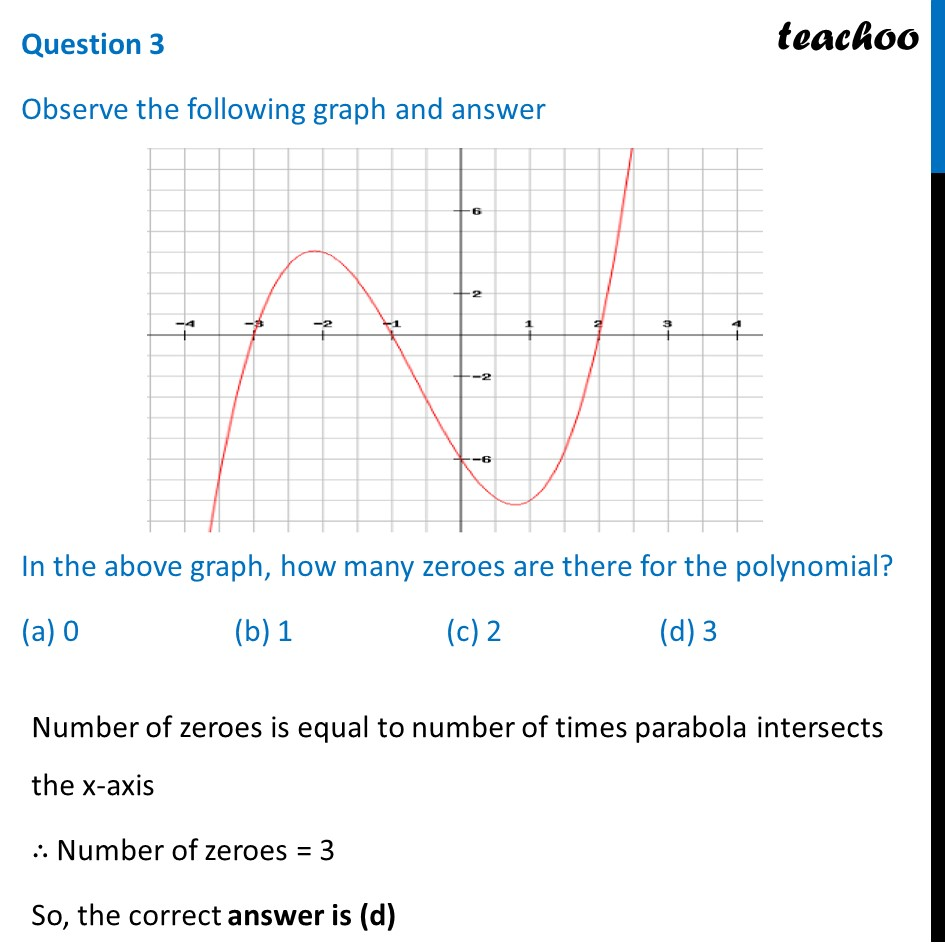## The three zeroes in the above shown graph are (a) 2, 3,–1   (b) –2, 3, 1  (c) –3, –1, 2   (d) – 2, –3, –1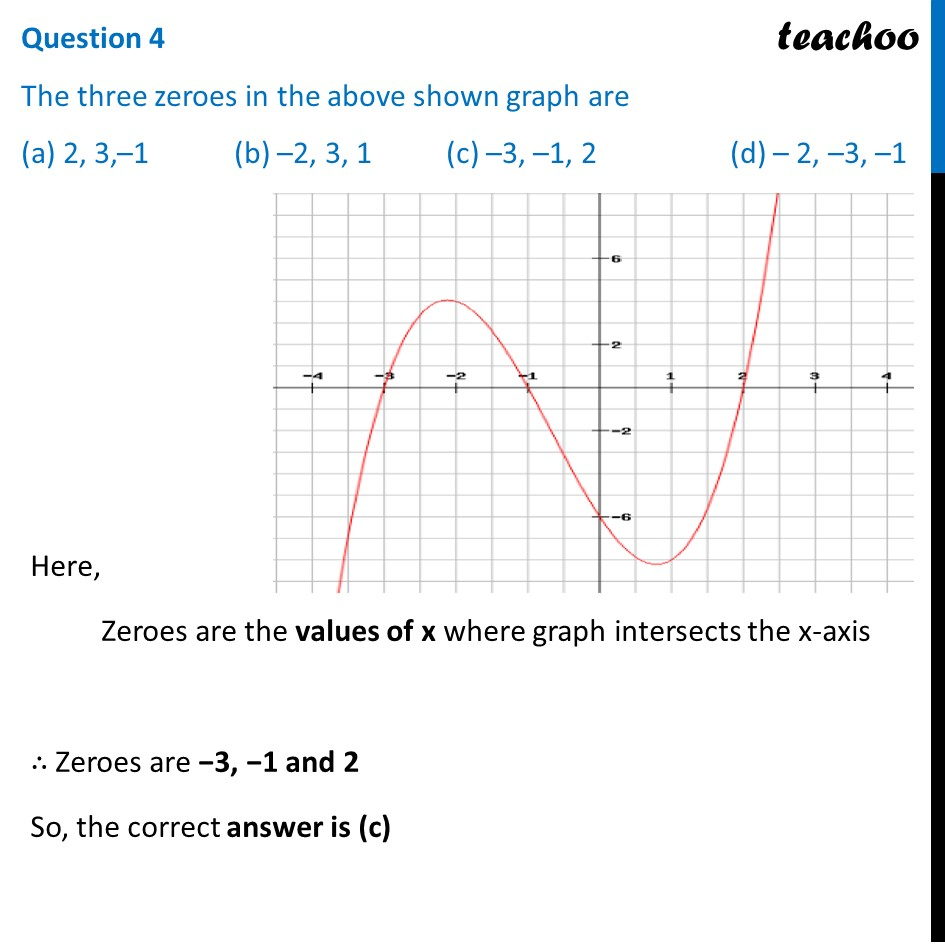## What will be the expression of the polynomial? (a) x 3 +2x 2 -5x-6  (b) x 3 +2x 2 -5x+6    (c) x 3 +2x 2 +5x-6 (d) x 3 +2x 2 +5x+6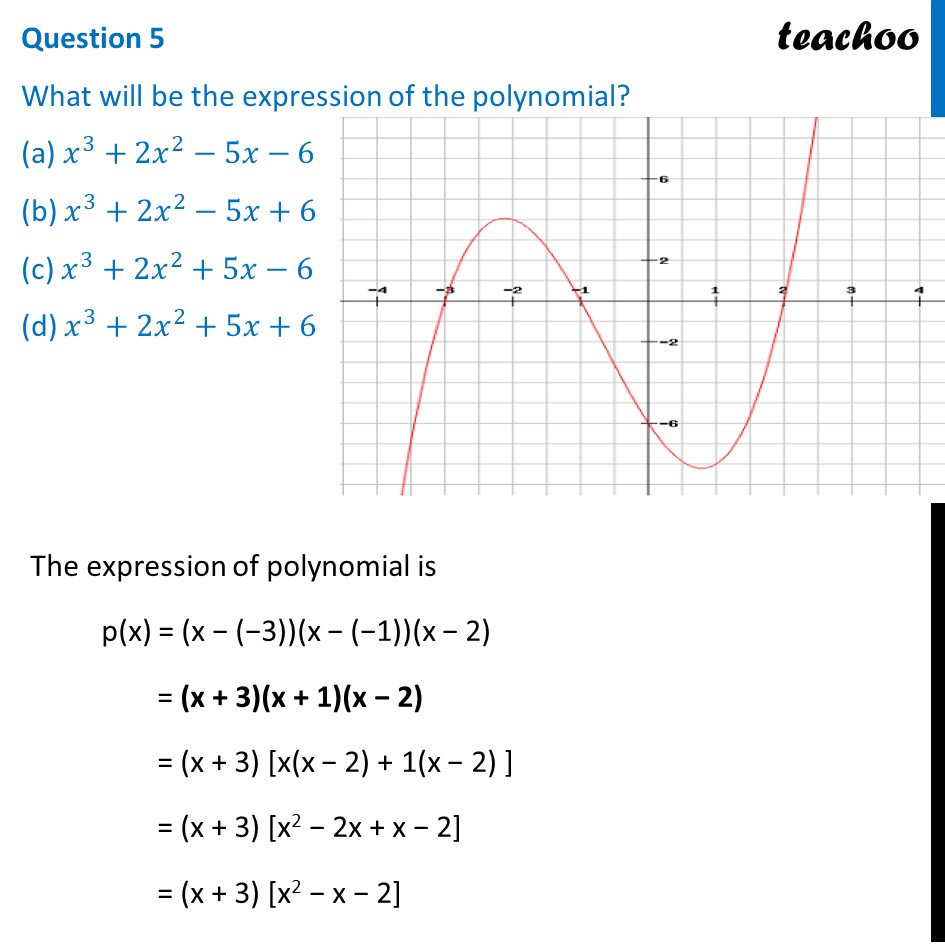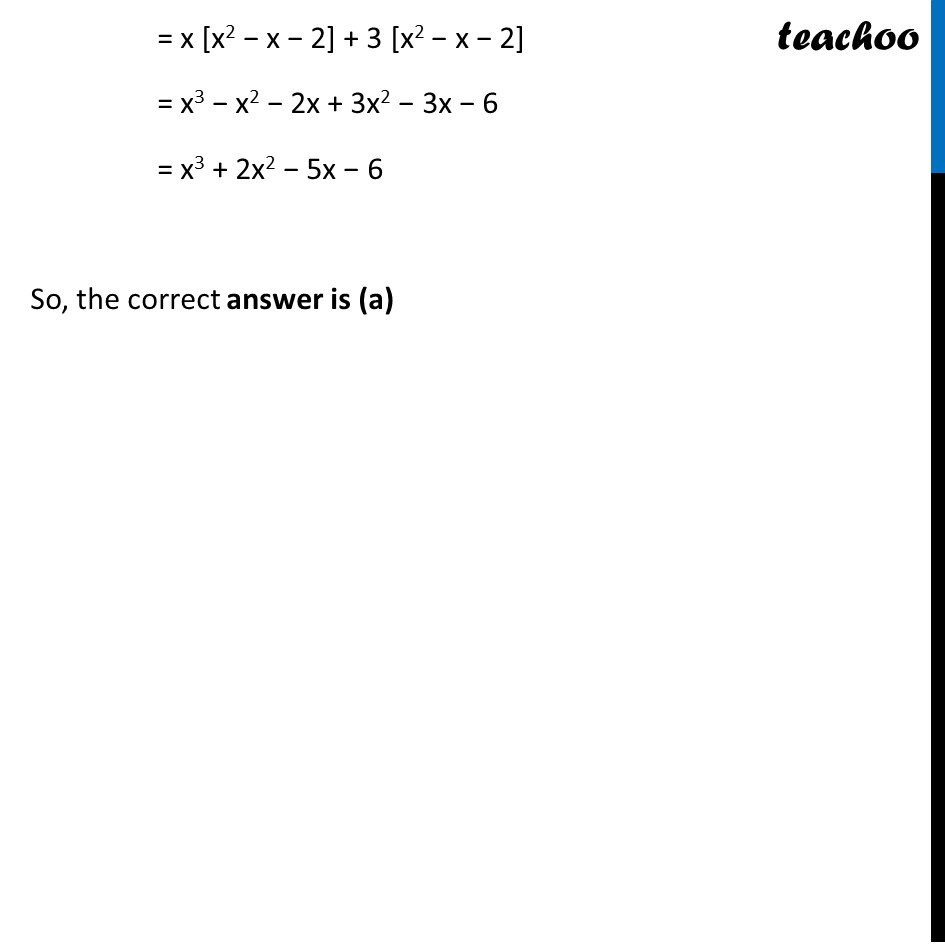1. Chapter 2 Class 10 Polynomials (Term 1)
2. Serial order wise
3. Case Based Questions (MCQ)

Transcript

Question Basketball and soccer are played with a spherical ball. Even though an athlete dribbles the ball in both sports, a basketball player uses his hands and a soccer player uses his feet. Usually, soccer is played outdoors on a large field and basketball is played indoor on a court made out of wood. The projectile (path traced) of soccer ball and basketball are in the form of parabola representing quadratic polynomialQuestion 1 The shape of the path traced shown is (a) Spiral (b) Ellipse (c) Linear (d) Parabola The shape of the path traced is a parabola So, the correct answer is (d) Question 2 The graph of parabola opens upwards, if _______ (a) a = 0 (b) a < 0 (c) a > 0 (d) a ≥ 0 For a quadratic polynomial ax2 + bx + c Here, a can never be 0 And if a > 0, then graph opens upwards Example: x2 + 2 So, the correct answer is (c) Question 3 Observe the following graph and answer In the above graph, how many zeroes are there for the polynomial? (a) 0 (b) 1 (c) 2 (d) 3 Number of zeroes is equal to number of times parabola intersects the x-axis ∴ Number of zeroes = 3 So, the correct answer is (d) Question 4 The three zeroes in the above shown graph are (a) 2, 3,–1 (b) –2, 3, 1 (c) –3, –1, 2 (d) – 2, –3, –1 Here, Zeroes are the values of x where graph intersects the x-axis ∴ Zeroes are −3, −1 and 2 So, the correct answer is (c) Question 5 What will be the expression of the polynomial? (a) 𝑥^3+2𝑥^2−5𝑥−6 (b) 𝑥^3+2𝑥^2−5𝑥+6 (c) 𝑥^3+2𝑥^2+5𝑥−6 (d) 𝑥^3+2𝑥^2+5𝑥+6 The expression of polynomial is p(x) = (x − (−3))(x − (−1))(x − 2) = (x + 3)(x + 1)(x − 2) = (x + 3) [x(x − 2) + 1(x − 2) ] = (x + 3) [x2 − 2x + x − 2] = (x + 3) [x2 − x − 2] = x [x2 − x − 2] + 3 [x2 − x − 2] = x3 − x2 − 2x + 3x2 − 3x − 6 = x3 + 2x2 − 5x − 6 So, the correct answer is (a)

Case Based Questions (MCQ)